# Category: absolute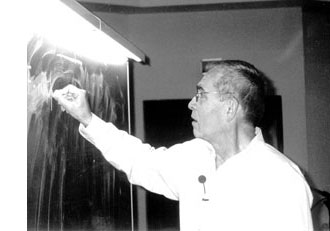Mumford’s drawing has a clear emphasis on the vertical direction. The set of all vertical lines corresponds to taking the fibers of the natural ‘structural morphism’ : $\pi~:~\mathbf{spec}(\mathbb{Z}[t]) \rightarrow \mathbf{spec}(\mathbb{Z})$ coming from the inclusion $\mathbb{Z} \subset \mathbb{Z}[t]$. That is, we consider the intersection $P \cap \mathbb{Z}$ of a prime ideal $P \subset \mathbb{Z}[t]$ with the subring of constants.

Two options arise : either $P \cap \mathbb{Z} \not= 0$, in which case the intersection is a principal prime ideal $~(p)$ for some prime number $p$ (and hence $P$ itself is bigger or equal to $p\mathbb{Z}[t]$ whence its geometric object is contained in the vertical line $\mathbb{V}((p))$, the fiber $\pi^{-1}((p))$ of the structural morphism over $~(p)$), or, the intersection $P \cap \mathbb{Z}[t] = 0$ reduces to the zero ideal (in which case the extended prime ideal $P \mathbb{Q}[x] = (q(x))$ is a principal ideal of the rational polynomial algebra $\mathbb{Q}[x]$, and hence the geometric object corresponding to $P$ is a horizontal curve in Mumford’s drawing, or is the whole arithmetic plane itself if $P=0$).

Because we know already that any ‘point’ in Mumford’s drawing corresponds to a maximal ideal of the form $\mathfrak{m}=(p,f(x))$ (see last time), we see that every point lies on precisely one of the set of all vertical coordinate axes corresponding to the prime numbers ${~\mathbb{V}((p)) = \mathbf{spec}(\mathbb{F}_p[x]) = \pi^{-1}((p))~}$. In particular, two different vertical lines do not intersect (or, in ringtheoretic lingo, the ‘vertical’ prime ideals $p\mathbb{Z}[x]$ and $q\mathbb{Z}[x]$ are comaximal for different prime numbers $p \not= q$).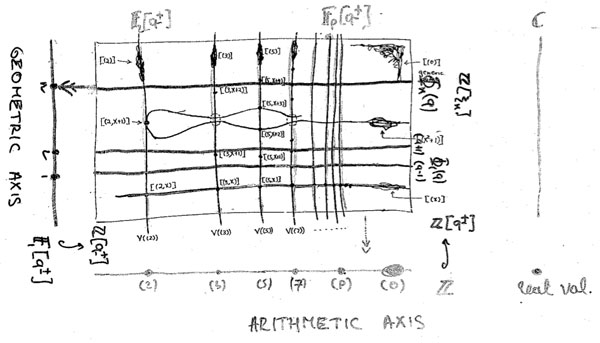That is, the structural morphism is a projection onto the “arithmetic axis” (which is $\mathbf{spec}(\mathbb{Z})$) and we get the above picture. The extra vertical line to the right of the picture is there because in arithmetic geometry it is customary to include also the archimedean valuations and hence to consider the ‘compactification’ of the arithmetic axis $\mathbf{spec}(\mathbb{Z})$ which is $\overline{\mathbf{spec}(\mathbb{Z})} = \mathbf{spec}(\mathbb{Z}) \cup { v_{\mathbb{R}} }$.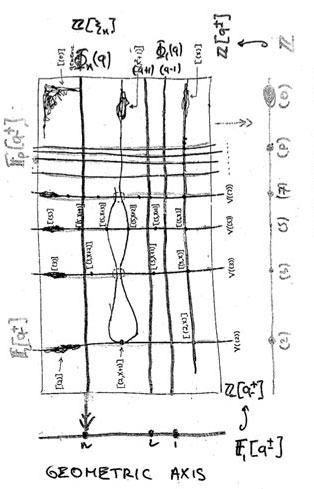Yuri I. Manin is advocating for years the point that we should take the terminology ‘arithmetic surface’ for $\mathbf{spec}(\mathbb{Z}[x])$ a lot more seriously. That is, there ought to be, apart from the projection onto the ‘z-axis’ (that is, the arithmetic axis $\mathbf{spec}(\mathbb{Z})$) also a projection onto the ‘x-axis’ which he calls the ‘geometric axis’.

But then, what are the ‘points’ of this geometric axis and what are their fibers under this second projection?

We have seen above that the vertical coordinate line over the prime number $~(p)$ coincides with $\mathbf{spec}(\mathbb{F}_p[x])$, the affine line over the finite field $\mathbb{F}_p$. But all of these different lines, for varying primes $p$, should project down onto the same geometric axis. Manin’s idea was to take therefore as the geometric axis the affine line $\mathbf{spec}(\mathbb{F}_1[x])$, over the virtual field with one element, which should be thought of as being the limit of the finite fields $\mathbb{F}_p$ when $p$ goes to one!

How many points does $\mathbf{spec}(\mathbb{F}_1[x])$ have? Over a virtual object one can postulate whatever one wants and hope for an a posteriori explanation. $\mathbb{F}_1$-gurus tell us that there should be exactly one point of size n on the affine line over $\mathbb{F}_1$, corresponding to the unique degree n field extension $\mathbb{F}_{1^n}$. However, it is difficult to explain this from the limiting perspective…

Over a genuine finite field $\mathbb{F}_p$, the number of points of thickness $n$ (that is, those for which the residue field is isomorphic to the degree n extension $\mathbb{F}_{p^n}$) is equal to the number of monic irreducible polynomials of degree n over $\mathbb{F}_p$. This number is known to be $\frac{1}{n} \sum_{d | n} \mu(\frac{n}{d}) p^d$ where $\mu(k)$ is the Moebius function. But then, the limiting number should be $\frac{1}{n} \sum_{d | n} \mu(\frac{n}{d}) = \delta_{n1}$, that is, there can only be one point of size one…

Alternatively, one might consider the zeta function counting the number $N_n$ of ideals having a quotient consisting of precisely $p^n$ elements. Then, we have for genuine finite fields $\mathbb{F}_p$ that $\zeta(\mathbb{F}_p[x]) = \sum_{n=0}^{\infty} N_n t^n = 1 + p t + p^2 t^2 + p^3 t^3 + \ldots$, whence in the limit it should become
$1+t+t^2 +t^3 + \ldots$ and there is exactly one ideal in $\mathbb{F}_1[x]$ having a quotient of cardinality n and one argues that this unique quotient should be the unique point with residue field $\mathbb{F}_{1^n}$ (though it might make more sense to view this as the unique n-fold extension of the unique size-one point $\mathbb{F}_1$ corresponding to the quotient $\mathbb{F}_1[x]/(x^n)$…)

A perhaps more convincing reasoning goes as follows. If $\overline{\mathbb{F}_p}$ is an algebraic closure of the finite field $\mathbb{F}_p$, then the points of the affine line over $\overline{\mathbb{F}_p}$ are in one-to-one correspondence with the maximal ideals of $\overline{\mathbb{F}_p}[x]$ which are all of the form $~(x-\lambda)$ for $\lambda \in \overline{\mathbb{F}_p}$. Hence, we get the points of the affine line over the basefield $\mathbb{F}_p$ as the orbits of points over the algebraic closure under the action of the Galois group $Gal(\overline{\mathbb{F}_p}/\mathbb{F}_p)$.

‘Common wisdom’ has it that one should identify the algebraic closure of the field with one element $\overline{\mathbb{F}_{1}}$ with the group of all roots of unity $\mathbb{\mu}_{\infty}$ and the corresponding Galois group $Gal(\overline{\mathbb{F}_{1}}/\mathbb{F}_1)$ as being generated by the power-maps $\lambda \rightarrow \lambda^n$ on the roots of unity. But then there is exactly one orbit of length n given by the n-th roots of unity $\mathbb{\mu}_n$, so there should be exactly one point of thickness n in $\mathbf{spec}(\mathbb{F}_1[x])$ and we should then identity the corresponding residue field as $\mathbb{F}_{1^n} = \mathbb{\mu}_n$.

Whatever convinces you, let us assume that we can identify the non-generic points of $\mathbf{spec}(\mathbb{F}_1[x])$ with the set of positive natural numbers ${ 1,2,3,\ldots }$ with $n$ denoting the unique size n point with residue field $\mathbb{F}_{1^n}$. Then, what are the fibers of the projection onto the geometric axis $\phi~:~\mathbf{spec}(\mathbb{Z}[x]) \rightarrow \mathbf{spec}(\mathbb{F}_1[x]) = { 1,2,3,\ldots }$?

These fibers should correspond to ‘horizontal’ principal prime ideals of $\mathbb{Z}[x]$. Manin proposes to consider $\phi^{-1}(n) = \mathbb{V}((\Phi_n(x)))$ where $\Phi_n(x)$ is the n-th cyclotomic polynomial. The nice thing about this proposal is that all closed points of $\mathbf{spec}(\mathbb{Z}[x])$ lie on one of these fibers!

Indeed, the residue field at such a point (corresponding to a maximal ideal $\mathfrak{m}=(p,f(x))$) is the finite field $\mathbb{F}_{p^n}$ and as all its elements are either zero or an $p^n-1$-th root of unity, it does lie on the curve determined by $\Phi_{p^n-1}(x)$.

As a consequence, the localization $\mathbb{Z}[x]_{cycl}$ of the integral polynomial ring $\mathbb{Z}[x]$ at the multiplicative system generated by all cyclotomic polynomials is a principal ideal domain (as all height two primes evaporate in the localization), and, the fiber over the generic point of $\mathbf{spec}(\mathbb{F}_1[x])$ is $\mathbf{spec}(\mathbb{Z}[x]_{cycl})$, which should be compared to the fact that the fiber of the generic point in the projection onto the arithmetic axis is $\mathbf{spec}(\mathbb{Q}[x])$ and $\mathbb{Q}[x]$ is the localization of $\mathbb{Z}[x]$ at the multiplicative system generated by all prime numbers).

Hence, both the vertical coordinate lines and the horizontal ‘lines’ contain all closed points of the arithmetic plane. Further, any such closed point $\mathfrak{m}=(p,f(x))$ lies on the intersection of a vertical line $\mathbb{V}((p))$ and a horizontal one $\mathbb{V}((\Phi_{p^n-1}(x)))$ (if $deg(f(x))=n$).
That is, these horizontal and vertical lines form a coordinate system, at least for the closed points of $\mathbf{spec}(\mathbb{Z}[x])$.

Still, there is a noticeable difference between the two sets of coordinate lines. The vertical lines do not intersect meaning that $p\mathbb{Z}[x]+q\mathbb{Z}[x]=\mathbb{Z}[x]$ for different prime numbers p and q. However, in general the principal prime ideals corresponding to the horizontal lines $~(\Phi_n(x))$ and $~(\Phi_m(x))$ are not comaximal when $n \not= m$, that is, these ‘lines’ may have points in common! This will lead to an exotic new topology on the roots of unity… (to be continued).

It is perhaps surprising that Alain Connes and Katia Consani, two icons of noncommutative geometry, restrict themselves to define commutative algebraic geometry over $\mathbb{F}_1$, the field with one element.

My guess of why they stop there is as good as anyone’s. Perhaps they felt that there is already enough noncommutativity in Soule’s gadget-approach (the algebra $\mathcal{A}_X$ as in this post may very well be noncommutative). Perhaps they were only interested in the Bost-Connes system which can be entirely encoded in their commutative $\mathbb{F}_1$-geometry. Perhaps they felt unsure as to what the noncommutative scheme of an affine noncommutative algebra might be. Perhaps …

Remains the fact that their approach screams for a noncommutative extension. Their basic object is a covariant functor

$N~:~\mathbf{abelian} \rightarrow \mathbf{sets} \qquad A \mapsto N(A)$

from finite abelian groups to sets, together with additional data to the effect that there is a unique minimal integral scheme associated to $N$. In a series of posts on the Connes-Consani paper (starting here) I took some care of getting rid of all scheme-lingo and rephrasing everything entirely into algebras. But then, this set-up can be extended verbatim to noncommuative $\mathbb{F}_1$-geometry, which should start from a covariant functor

$N~:~\mathbf{groups} \rightarrow \mathbf{sets}$

from all finite groups to sets. Let’s recall quickly what the additional info should be making this functor a noncommutative (affine) F_un scheme :

There should be a finitely generated $\mathbb{C}$-algebra $R$ together with a natural transformation (the ‘evaluation’)

$e~:~N \rightarrow \mathbf{maxi}(R) \qquad N(G) \mapsto Hom_{\mathbb{C}-alg}(R, \mathbb{C} G)$

(both $R$ and the group-algebra $\mathbb{C} G$ may be noncommutative). The pair $(N, \mathbf{maxi}(R))$ is then called a gadget and there is an obvious notion of ‘morphism’ between gadgets.

The crucial extra ingredient is an affine $\mathbb{Z}$-algebra (possibly noncommutative) $S$
such that $N$ is a subfunctor of $\mathbf{mini}(S)~:~G \mapsto Hom_{\mathbb{Z}-alg}(S,\mathbb{Z} G)$ together with the following universal property :

any affine $\mathbb{Z}$-algebra $T$ having a gadget-morphism $~(N,\mathbf{maxi}(R)) \rightarrow (\mathbf{mini}(T),\mathbf{maxi}(T \otimes_{\mathbb{Z}} \mathbb{C}))$ comes from a $\mathbb{Z}$-algebra morphism $T \rightarrow S$. (If this sounds too cryptic for you, please read the series on C-C mentioned before).

So, there is no problem in defining noncommutative affine F_un-schemes. However, as with any generalization, this only makes sense provided (a) we get something new and (b) we have interesting examples, not covered by the restricted theory.

At first sight we do not get something new as in the only example we did in the C-C-series (the forgetful functor) it is easy to prove (using the same proof as given in this post) that the forgetful-functor $\mathbf{groups} \rightarrow \mathbf{sets}$ still has as its integral form the integral torus $\mathbb{Z}[x,x^{-1}]$. However, both theories quickly diverge beyond this example.

For example, consider the functor

$\mathbf{groups} \rightarrow \mathbf{sets} \qquad G \mapsto G \times G$

Then, if we restrict to abelian finite groups $\mathbf{abelian}$ it is easy to see (again by a similar argument) that the two-dimensional integer torus $\mathbb{Z}[x,y,x^{-1},y^{-1}]$ is the correct integral form. However, this algebra cannot be the correct form for the functor on the category of all finite groups as any $\mathbb{Z}$-algebra map $\phi~:~\mathbb{Z}[x,y,x^{-1},y^{-1}] \rightarrow \mathbb{Z} G$ determines (and is determined by) a pair of commuting units in $\mathbb{Z} G$, so the above functor can not be a subfunctor if we allow non-Abelian groups.

But then, perhaps there isn’t a minimal integral $\mathbb{Z}$-form for this functor? Well, yes there is. Take the free group in two letters (that is, all words in noncommuting $x,y,x^{-1}$ and $y^{-1}$ satisfying only the trivial cancellation laws between a letter and its inverse), then the corresponding integral group-algebra $\mathbb{Z} \mathcal{F}_2$ does the trick.

Again, the proof-strategy is the same. Given a gadget-morphism we have an algebra map $f~:~T \mapsto \mathbb{C} \mathcal{F}_2$ and we have to show, using the universal property that the image of $T$ is contained in the integral group-algebra $\mathbb{Z} \mathcal{F}_2$. Take a generator
$z$ of $T$ then the degree of the image $f(z)$ is bounded say by $d$ and we can always find a subgroup $H \subset \mathcal{F}_2$ such that $\mathcal{F}_2/H$ is a fnite group and the quotient map $\mathbb{C} \mathcal{F}_2 \rightarrow \mathbb{C} \mathcal{F}_2/H$ is injective on the subspace spanned by all words of degree strictly less than $d+1$. Then, the usual diagram-chase finishes the proof.

What makes this work is that the free group $\mathcal{F}_2$ has ‘enough’ subgroups of finite index, a property it shares with many interesting discrete groups. Whence the blurb-message :

if the integers $\mathbb{Z}$ see a discrete group $\Gamma$, then the field $\mathbb{F}_1$ sees its profinite completion $\hat{\Gamma} = \underset{\leftarrow}{lim}~\Gamma/ H$So, yes, we get something new by extending the Connes-Consani approach to the noncommutative world, but do we have interesting examples? As “interesting” is a subjective qualification, we’d better invoke the authority-argument.

Alexander Grothendieck (sitting on the right, manifestly not disputing a vacant chair with Jean-Pierre Serre, drinking on the left (a marvelous picture taken by F. Hirzebruch in 1958)) was pushing the idea that profinite completions of arithmetical groups were useful in the study of the absolute Galois group $Gal(\overline{\mathbb{Q}}/\mathbb{Q})$, via his theory of dessins d’enfants (children;s drawings).

In a previous life, I’ve written a series of posts on dessins d’enfants, so I’ll restrict here to the basics. A smooth projective $\overline{\mathbb{Q}}$-curve $X$ has a Belyi-map $X \rightarrow \mathbb{P}^1_{\overline{\mathbb{Q}}}$ ramified only in three points ${ 0,1,\infty }$. The “drawing” corresponding to $X$ is a bipartite graph, drawn on the Riemann surface $X_{\mathbb{C}}$ obtained by lifting the unit interval $[0,1]$ to $X$. As the absolute Galois group acts on all such curves (and hence on their corresponding drawings), the action of it on these dessins d’enfants may give us a way into the multiple mysteries of the absolute Galois group.

In his “Esquisse d’un programme” (Sketch of a program if you prefer to read it in English) he writes :

“C’est ainsi que mon attention s’est portée vers ce que j’ai appelé depuis la “géométrie algêbrique anabélienne”, dont le point de départ est justement une étude (pour le moment limitée à la caractéristique zéro) de l’action de groupe de Galois “absolus” (notamment les groupes $Gal(\overline{K}/K)$, ou $K$ est une extension de type fini du corps premier) sur des groupes fondamentaux géométriques (profinis) de variétés algébriques (définies sur $K$), et plus particulièrement (rompant avec une tradition bien enracinée) des groupes fondamentaux qui sont trés éloignés des groupes abéliens (et que pour cette raison je nomme “anabéliens”). Parmi ces groupes, et trés proche du groupe $\hat{\pi}_{0,3}$, il y a le compactifié profini du groupe modulaire $SL_2(\mathbb{Z})$, dont le quotient par le centre $\pm 1$ contient le précédent comme sous-groupe de congruence mod 2, et peut s’interpréter d’ailleurs comme groupe “cartographique” orienté, savoir celui qui classifie les cartes orientées triangulées (i.e. celles dont les faces des triangles ou des monogones).”

and a bit further, he writes :

“L’élément de structure de $SL_2(\mathbb{Z})$ qui me fascine avant tout, est bien sur l’action extérieure du groupe de Galois $Gal(\overline{\mathbb{Q}}/\mathbb{Q})$ sur le compactifié profini. Par le théorème de Bielyi, prenant les compactifiés profinis de sous-groupes d’indice fini de $SL_2(\mathbb{Z})$, et l’action extérieure induite (quitte à passer également à un sous-groupe overt de $Gal(\overline{\mathbb{Q}},\mathbb{Q})$), on trouve essentiellement les groupes fondamentaux de toutes les courbes algébriques définis sur des corps de nombres $K$, et l’action extérieure de $Gal(\overline{K}/K)$ dessus.”

So, is there a noncommutative affine variety over $\mathbb{F}_1$ of which the unique minimal integral model is the integral group algebra of the modular group $\mathbb{Z} \Gamma$ (with $\Gamma = PSL_2(\mathbb{Z})$? Yes, here it is

$N_{\Gamma}~:~\mathbf{groups} \rightarrow \mathbf{sets} \qquad G \mapsto G_2 \times G_3$

where $G_n$ is the set of all elements of order $n$ in $G$. The reason behind this is that the modular group is the free group product $C_2 \ast C_3$.

Fine, you may say, but all this is just algebra. Where is the noncommutative complex variety or the noncommutative integral scheme in all this? Well, we can introduce them too but as this post is already 1300 words long, I’ll better leave this for another time. In case you cannot stop thinking about it, here’s the short answer.

The complex noncommutative variety has as its ‘points’ all finite dimensional simple complex representations of the modular group, and the ‘points’ of the noncommutative $\mathbb{F}_1$-scheme are exactly the (modular) dessins d’enfants…

In case you haven’t noticed it yet : I’m not living here anymore.

My blogging is (at least for the moment) transfered to the F_un Mathematics blog which some prefer to call the “ceci n’est pas un corps”-blog, which is very fine with me.

Javier gave a talk at MPI on Soule’s approach to algebraic geometry over the elusive field with one element $\mathbb{F}_1$ and wrote two posts about it The skeleton of Soule’s F_un geometry and Gadgets a la Soule. The rough idea being that a variety over the field with one element only acquires flesh after a base extension to $\mathbb{Z}$ and to cyclotomic integers.

I did some posts on a related (but conceptually somewhat easier) approach due to Alain Connes and Katia Consani. I’ve tried to explain their construction at the level of (mature) undergraduate students. So far, there are three posts part1, part2 and part3. Probably there is one more session to come in which I will explain why they need functors to graded sets.

In the weeks to come we plan to post about applications of this F_un-geometry to noncommutative geometry (the Bost-Connes system) and Grothendieck’s anabelian geometry (the theory of dessins d’enfant). I’ll try to leave a short account of the main posts here, but clearly you are invited to feed your feedreader this.

Perhaps I’ll return here for a week mid november to do some old-fashioned vacation blogging. I have to admit I did underestimate Numeo.fr. Rumours have it that our place is connected wirelessly to the web…

At the Max-Planck Institute in Bonn Yuri Manin gave a talk about the field of one element, $\mathbb{F}_1$ earlier this week entitled “Algebraic and analytic geometry over the field F_1”.

Moreover, Javier Lopez-Pena and Bram Mesland will organize a weekly “F_un Study Seminar” starting next tuesday.

Over at Noncommutative Geometry there is an Update on the field with one element pointing us to a YouTube-clip featuring Alain Connes explaining his paper with Katia Consani and Matilde Marcolli entitled “Fun with F_un”. Here’s the clip

Finally, as I’ll be running a seminar here too on F_un, we’ve set up a group blog with the people from MPI (clearly, if you are interested to join us, just tell!). At the moment there are just a few of my old F_un posts and a library of F_un papers, but hopefully a lot will be added soon. So, have a look at F_un mathematics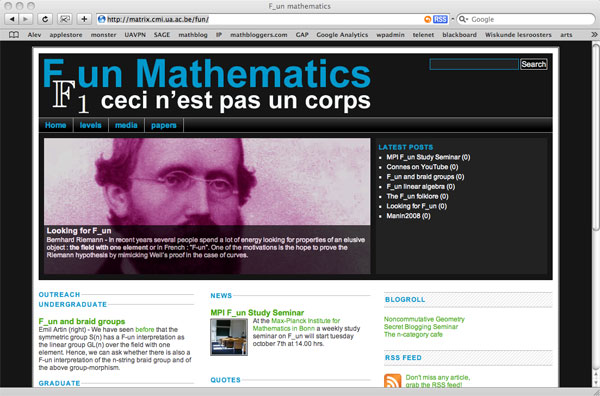A couple of weeks ago, Alain Connes and Katia Consani arXived their paper “On the notion of geometry over $\mathbb{F}_1$”. Their subtle definition is phrased entirely in Grothendieck‘s scheme-theoretic language of representable functors and may be somewhat hard to get through if you only had a few years of mathematics.I’ll try to give the essence of their definition of an affine scheme over $\mathbb{F}_1$ (and illustrate it with an example) in a couple of posts. All you need to know is what a finite Abelian group is (if you know what a cyclic group is that’ll be enough) and what a commutative algebra is. If you already know what a functor and a natural transformation is, that would be great, but we’ll deal with all that abstract nonsense when we’ll need it.

So take two finite Abelian groups A and B, then a group-morphism is just a map $f~:~A \rightarrow B$ preserving the group-data. That is, f sends the unit element of A to that of B and
f sends a product of two elements in A to the product of their images in B. For example, if $A=C_n$ is a cyclic group of order n with generator g and $B=C_m$ is a cyclic group of order m with generator h, then every groupmorphism from A to B is entirely determined by the image of g let’s say that this image is $h^i$. But, as $g^n=1$ and the conditions on a group-morphism we must have that $h^{in} = (h^i)^n = 1$ and therefore m must divide i.n. This gives you all possible group-morphisms from A to B.

They are plenty of finite abelian groups and many group-morphisms between any pair of them and all this stuff we put into one giant sack and label it $\mathbf{abelian}$. There is another, even bigger sack, which is even simpler to describe. It is labeled $\mathbf{sets}$ and contains all sets as well as all maps between two sets.

Right! Now what might be a map $F~:~\mathbf{abelian} \rightarrow \mathbf{sets}$ between these two sacks? Well, F should map any abelian group A to a set F(A) and any group-morphism $f~:~A \rightarrow B$ to a map between the corresponding sets $F(f)~:~F(A) \rightarrow F(B)$ and do all of this nicely. That is, F should send compositions of group-morphisms to compositions of the corresponding maps, and so on. If you take a pen and a piece of paper, you’re bound to come up with the exact definition of a functor (that’s what F is called).

You want an example? Well, lets take F to be the map sending an Abelian group A to its set of elements (also called A) and which sends a groupmorphism $A \rightarrow B$ to the same map from A to B. All F does is ‘forget’ the extra group-conditions on the sets and maps. For this reason F is called the forgetful functor. We will denote this particular functor by $\underline{\mathbb{G}}_m$, merely to show off.

Luckily, there are lots of other and more interesting examples of such functors. Our first class we will call maxi-functors and they are defined using a finitely generated $\mathbb{C}$-algebra R. That is, R can be written as the quotient of a polynomial algebra

$R = \frac{\mathbb{C}[x_1,\ldots,x_d]}{(f_1,\ldots,f_e)}$

by setting all the polynomials $f_i$ to be zero. For example, take R to be the ring of Laurant polynomials

$R = \mathbb{C}[x,x^{-1}] = \frac{\mathbb{C}[x,y]}{(xy-1)}$

Other, and easier, examples of $\mathbb{C}$-algebras is the group-algebra $\mathbb{C} A$ of a finite Abelian group A. This group-algebra is a finite dimensional vectorspace with basis $e_a$, one for each element $a \in A$ with multiplication rule induced by the relations $e_a.e_b = e_{a.b}$ where on the left-hand side the multiplication . is in the group-algebra whereas on the right hand side the multiplication in the index is that of the group A. By choosing a different basis one can show that the group-algebra is really just the direct sum of copies of $\mathbb{C}$ with component-wise addition and multiplication

$\mathbb{C} A = \mathbb{C} \oplus \ldots \oplus \mathbb{C}$

with as many copies as there are elements in the group A. For example, for the cyclic group $C_n$ we have

$\mathbb{C} C_n = \frac{\mathbb{C}[x]}{(x^n-1)} = \frac{\mathbb{C}[x]}{(x-1)} \oplus \frac{\mathbb{C}[x]}{(x-\zeta)} \oplus \frac{\mathbb{C}[x]}{(x-\zeta^2)} \oplus \ldots \oplus \frac{\mathbb{C}[x]}{(x-\zeta^{n-1})} = \mathbb{C} \oplus \mathbb{C} \oplus \mathbb{C} \oplus \ldots \oplus \mathbb{C}$

The maxi-functor asociated to a $\mathbb{C}$-algebra R is the functor

$\mathbf{maxi}(R)~:~\mathbf{abelian} \rightarrow \mathbf{sets}$

which assigns to a finite Abelian group A the set of all algebra-morphism $R \rightarrow \mathbb{C} A$ from R to the group-algebra of A. But wait, you say (i hope), we also needed a functor to do something on groupmorphisms $f~:~A \rightarrow B$. Exactly, so to f we have an algebra-morphism $f’~:~\mathbb{C} A \rightarrow \mathbb{C}B$ so the functor on morphisms is defined via composition

$\mathbf{maxi}(R)(f)~:~\mathbf{maxi}(R)(A) \rightarrow \mathbf{maxi}(R)(B) \qquad \phi~:~R \rightarrow \mathbb{C} A \mapsto f’ \circ \phi~:~R \rightarrow \mathbb{C} A \rightarrow \mathbb{C} B$

So, what is the maxi-functor $\mathbf{maxi}(\mathbb{C}[x,x^{-1}]$? Well, any $\mathbb{C}$-algebra morphism $\mathbb{C}[x,x^{-1}] \rightarrow \mathbb{C} A$ is fully determined by the image of $x$ which must be a unit in $\mathbb{C} A = \mathbb{C} \oplus \ldots \oplus \mathbb{C}$. That is, all components of the image of $x$ must be non-zero complex numbers, that is

$\mathbf{maxi}(\mathbb{C}[x,x^{-1}])(A) = \mathbb{C}^* \oplus \ldots \oplus \mathbb{C}^*$

where there are as many components as there are elements in A. Thus, the sets $\mathbf{maxi}(R)(A)$ are typically huge which is the reason for the maxi-terminology.

Next, let us turn to mini-functors. They are defined similarly but this time using finitely generated $\mathbb{Z}$-algebras such as $S=\mathbb{Z}[x,x^{-1}]$ and the integral group-rings $\mathbb{Z} A$ for finite Abelian groups A. The structure of these inegral group-rings is a lot more delicate than in the complex case. Let’s consider them for the smallest cyclic groups (the ‘isos’ below are only approximations!)

$\mathbb{Z} C_2 = \frac{\mathbb{Z}[x]}{(x^2-1)} = \frac{\mathbb{Z}[x]}{(x-1)} \oplus \frac{\mathbb{Z}[x]}{(x+1)} = \mathbb{Z} \oplus \mathbb{Z}$

$\mathbb{Z} C_3 = \frac{\mathbb{Z}[x]}{(x^3-1)} = \frac{\mathbb{Z}[x]}{(x-1)} \oplus \frac{\mathbb{Z}[x]}{(x^2+x+1)} = \mathbb{Z} \oplus \mathbb{Z}[\rho]$

$\mathbb{Z} C_4 = \frac{\mathbb{Z}[x]}{(x^4-1)} = \frac{\mathbb{Z}[x]}{(x-1)} \oplus \frac{\mathbb{Z}[x]}{(x+1)} \oplus \frac{\mathbb{Z}[x]}{(x^2+1)} = \mathbb{Z} \oplus \mathbb{Z} \oplus \mathbb{Z}[i]$

For a $\mathbb{Z}$-algebra S we can define its mini-functor to be the functor

$\mathbf{mini}(S)~:~\mathbf{abelian} \rightarrow \mathbf{sets}$

which assigns to an Abelian group A the set of all $\mathbb{Z}$-algebra morphisms $S \rightarrow \mathbb{Z} A$. For example, for the algebra $\mathbb{Z}[x,x^{-1}]$ we have that

$\mathbf{mini}(\mathbb{Z} [x,x^{-1}]~(A) = (\mathbb{Z} A)^*$

the set of all invertible elements in the integral group-algebra. To study these sets one has to study the units of cyclotomic integers. From the above decompositions it is easy to verify that for the first few cyclic groups, the corresponding sets are $\pm C_2, \pm C_3$ and $\pm C_4$. However, in general this set doesn’t have to be finite. It is a well-known result that the group of units of an integral group-ring of a finite Abelian group is of the form

$(\mathbb{Z} A)^* = \pm A \times \mathbb{Z}^{\oplus r}$

where $r = \frac{1}{2}(o(A) + 1 + n_2 -2c)$ where $o(A)$ is the number of elements of A, $n_2$ is the number of elements of order 2 and c is the number of cyclic subgroups of A. So, these sets can still be infinite but at least they are a lot more manageable, explaining the mini-terminology.

Now, we would love to go one step deeper and define nano-functors by the same procedure, this time using finitely generated algebras over $\mathbb{F}_1$, the field with one element. But as we do not really know what we might mean by this, we simply define a nano-functor to be a subfunctor of a mini-functor, that is, a nano-functor N has an associated mini-functor $\mathbf{mini}(S)$ such that for all finite Abelian groups A we have that $N(A) \subset \mathbf{mini}(S)(A)$.

For example, the forgetful functor at the beginning, which we pompously denoted $\underline{\mathbb{G}}_m$ is a nano-functor as it is a subfunctor of the mini-functor $\mathbf{mini}(\mathbb{Z}[x,x^{-1}])$.

Now we are allmost done : an affine $\mathbb{F}_1$-scheme in the sense of Connes and Consani is a pair consisting of a nano-functor N and a maxi-functor $\mathbf{maxi}(R)$ such that two rather strong conditions are satisfied :

• there is an evaluation ‘map’ of functors $e~:~N \rightarrow \mathbf{maxi}(R)$
• this pair determines uniquely a ‘minimal’ mini-functor $\mathbf{mini}(S)$ of which N is a subfunctor

of course we still have to turn this into proper definitions but that will have to await another post. For now, suffice it to say that the pair $~(\underline{\mathbb{G}}_m,\mathbf{maxi}(\mathbb{C}[x,x^{-1}]))$ is a $\mathbb{F}_1$-scheme with corresponding uniquely determined mini-functor $\mathbf{mini}(\mathbb{Z}[x,x^{-1}])$, called the multiplicative group scheme.

Continued here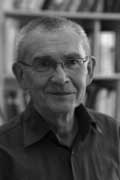Amidst all LHC-noise, Yuri I. Manin arXived today an interesting paper Cyclotomy and analytic geometry over $\mathbb{F}_1$.

The paper gives a nice survey of the existent literature and focusses on the crucial role of roots of unity in the algebraic geometry over the non-existent field with one element $\mathbb{F}_1$ (in French called ‘F-un’). I have tried to do a couple of posts on F-un some time ago but now realize, reading Manin’s paper, I may have given up way too soon…

At several places in the paper, Manin hints at a possible noncommutative geometry over $\mathbb{F}_1$ :

This is the appropriate place to stress that in a wider context of Toen-Vaqui ‘Au-dessous de Spec Z’, or eventually in noncommutative $\mathbb{F}_1$-geometry, teh spectrum of $\mathbb{F}_1$ loses its privileged position as a final object of a geometric category. For example, in noncommutative geometry, or in an appropriate category of stacks, the quotient of this spectrum modulo the trivial action of a group must lie below this spectrum.

Soule’s algebras $\mathcal{A}_X$ are a very important element of the structure, in particular, because they form a bridge to Arakelov geometry. Soule uses concrete choices of them in order to produce ‘just right’ supply of morphisms, without a priori constraining these choices formally. In this work, we use these algebras and their version also to pave a way to the analytic (and possibly non-commutative) geometry over $\mathbb{F}_1$.

Back when I was writing the first batch of F-un posts, I briefly contemplated the possibility of a noncommutative geometry over $\mathbb{F}_1$, but quickly forgot about it because I thought it would be forced to reduce to commutative geometry.

Here is the quick argument : noncommutative geometry is really the study of coalgebras (see for example my paper or if you prefer more trustworthy sources the Kontsevich-Soibelman paper). Now, unless I made a mistake, I think all coalgebras over $\mathbb{F}_1$ must be co-commutative (even group-like), so reducing to commutative geometry.

Surely, I’m missing something…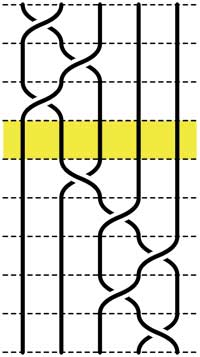Recall that an n-braid consists of n strictly descending elastic strings connecting n inputs at the top (named 1,2,…,n) to n outputs at the bottom (labeled 1,2,…,n) upto isotopy (meaning that we may pull and rearrange the strings in any way possible within 3-dimensional space). We can always change the braid slightly such that we can divide the interval between in- and output in a number of subintervals such that in each of those there is at most one crossing.

n-braids can be multiplied by putting them on top of each other and connecting the outputs of the first braid trivially to the inputs of the second. For example the 5-braid on the left can be written as $B=B_1.B_2$ with $B_1$ the braid on the top 3 subintervals and $B_2$ the braid on the lower 5 subintervals.

In this way (and using our claim that there can be at most 1 crossing in each subinterval) we can write any n-braid as a word in the generators $\sigma_i$ (with $1 \leq i < n$) being the overcrossing between inputs i and i+1. Observe that the undercrossing is then the inverse $\sigma_i^{-1}$. For example, the braid on the left corresponds to the word

$\sigma_1^{-1}.\sigma_2^{-1}.\sigma_1^{-1}.\sigma_2.\sigma_3^{-1}.\sigma_4^{-1}.\sigma_3^{-1}.\sigma_4$

Clearly there are relations among words in the generators. The easiest one we have already used implicitly namely that $\sigma_i.\sigma_i^{-1}$ is the trivial braid. Emil Artin proved in the 1930-ies that all such relations are consequences of two sets of ‘obvious’ relations. The first being commutation relations between crossings when the strings are far enough from each other. That is we have

$\sigma_i . \sigma_j = \sigma_j . \sigma_i$ whenever $|i-j| \geq 2$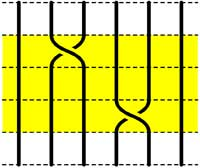=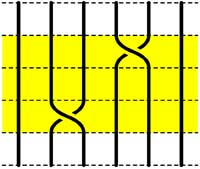The second basic set of relations involves crossings using a common string

$\sigma_i.\sigma_{i+1}.\sigma_i = \sigma_{i+1}.\sigma_i.\sigma_{i+1}$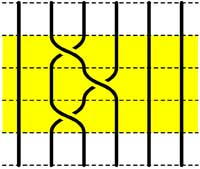=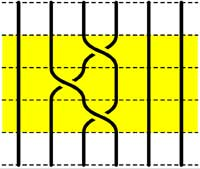Starting with the 5-braid at the top, we can use these relations to reduce it to a simpler form. At each step we have outlined to region where the relations are applied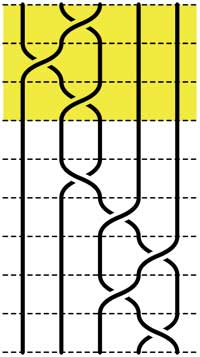=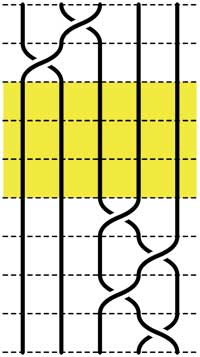=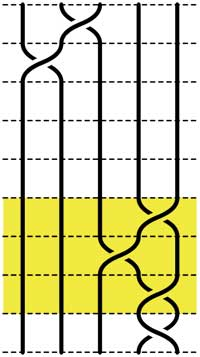=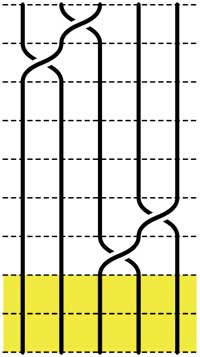These beautiful braid-pictures were produced using the braid-metapost program written by Stijn Symens.

Tracing a string from an input to an output assigns to an n-braid a permutation on n letters. In the above example, the permutation is $~(1,2,4,5,3)$. As this permutation doesn’t change under applying basic reduction, this gives a group-morphism

$\mathbb{B}_n \rightarrow S_n$

from the braid group on n strings $\mathbb{B}_n$ to the symmetric group. We have seen before that the symmetric group $S_n$ has a F-un interpretation as the linear group $GL_n(\mathbb{F}_1)$ over the field with one element. Hence, we can ask whether there is also a F-un interpretation of the n-string braid group and of the above group-morphism.

Kapranov and Smirnov suggest in their paper that the n-string braid group $\mathbb{B}_n \simeq GL_n(\mathbb{F}_1[t])$ is the general linear group over the polynomial ring $\mathbb{F}_1[t]$ over the field with one element and that the evaluation morphism (setting t=0)

$GL_n(\mathbb{F}_1[t]) \rightarrow GL_n(\mathbb{F}1)$ gives the groupmorphism $\mathbb{B}_n \rightarrow S_n$

The rationale behind this analogy is a theorem of Drinfeld‘s saying that over a finite field $\mathbb{F}_q$, the profinite completion of $GL_n(\mathbb{F}_q[t])$ is embedded in the fundamental group of the space of q-polynomials of degree n in much the same way as the n-string braid group $\mathbb{B}_n$ is the fundamental group of the space of complex polynomials of degree n without multiple roots.

And, now that we know the basics of absolute linear algebra, we can give an absolute braid-group representation

$\mathbb{B}_n = GL_n(\mathbb{F}_1[t]) \rightarrow GL_n(\mathbb{F}_{1^n})$

obtained by sending each generator $\sigma_i$ to the matrix over $\mathbb{F}_{1^n}$ (remember that $\mathbb{F}_{1^n} = (\mu_n)^{\bullet}$ where $\mu_n = \langle \epsilon_n \rangle$ are the n-th roots of unity)

$\sigma_i \mapsto \begin{bmatrix} 1_{i-1} & & & \\ & 0 & \epsilon_n & \\ & \epsilon_n^{-1} & 0 & \\ & & & 1_{n-1-i} \end{bmatrix}$

and it is easy to see that these matrices do indeed satisfy Artin’s defining relations for $\mathbb{B}_n$.

Today we will define some basic linear algebra over the absolute fields $\mathbb{F}_{1^n}$ following the Kapranov-Smirnov document. Recall from last time that $\mathbb{F}_{1^n} = \mu_n^{\bullet}$ and that a d-dimensional vectorspace over this field is a pointed set $V^{\bullet}$ where $V$ is a free $\mu_n$-set consisting of n.d elements. Note that in absolute linear algebra we are not allowed to have addition of vectors and have to define everything in terms of scalar multiplication (or if you want, the $\mu_n$-action). In the hope of keeping you awake, we will include an F-un interpretation of the power residue symbol.

Direct sums of vectorspaces are defined via $V^{\bullet} \oplus W^{\bullet} = (V \bigsqcup W)^{\bullet}$, that is, correspond to the disjoint union of free $\mu_n$-sets. Consequently we have that $dim(V^{\bullet} \oplus W^{\bullet}) = dim(V^{\bullet}) + dim(W^{\bullet})$.

For tensor-product we start with $V^{\bullet} \times W^{\bullet} = (V \times W)^{\bullet}$ the vectorspace cooresponding to the Cartesian product of free $\mu_n$-sets. If the dimensions of $V^{\bullet}$ and $W^{\bullet}$ are respectively d and e, then $V \times W$ consists of n.d.n.e elements, so is of dimension n.d.e. In order to have a sensible notion of tensor-products we have to eliminate the n-factor. We do this by identifying $~(x,y)$ with $(\epsilon_n x, \epsilon^{-1} y)$ and call the corresponding vectorspace $V^{\bullet} \otimes W^{\bullet}$. If we denote the image of $~(x,y)$ by $x \otimes w$ then the identification merely says we can pull the $\mu_n$-action through the tensor-sign, as we’d like to do. With this definition we do indeed have that $dim(V^{\bullet} \otimes W^{\bullet}) = dim(V^{\bullet}) dim(W^{\bullet})$.

Recall that any linear automorphism $A$ of an $\mathbb{F}_{1^n}$ vectorspace $V^{\bullet}$ with basis ${ b_1,\ldots,b_d }$ (representants of the different $\mu_n$-orbits) is of the form $A(b_i) = \epsilon_n^{k_i} b_{\sigma(i)}$ for some powers of the primitive n-th root of unity $\epsilon_n$ and some permutation $\sigma \in S_d$. We define the determinant $det(A) = \prod_{i=1}^d \epsilon_n^{k_i}$. One verifies that the determinant is multiplicative and independent of the choice of basis.

For example, scalar-multiplication by $\epsilon_n$ gives an automorphism on any $d$-dimensional $\mathbb{F}_{1^n}$-vectorspace $V^{\bullet}$ and the corresponding determinant clearly equals $det = \epsilon_n^d$. That is, the det-functor remembers the dimension modulo n. These mod-n features are a recurrent theme in absolute linear algebra. Another example, which will become relevant when we come to reciprocity laws :

Take $n=2$. Then, a $\mathbb{F}_{1^2}$ vectorspace $V^{\bullet}$ of dimension d is a set consisting of 2d elements $V$ equipped with a free involution. Any linear automorphism $A~:~V^{\bullet} \rightarrow V^{\bullet}$ is represented by a $d \times d$ matrix having one nonzero entry in every row and column being equal to +1 or -1. Hence, the determinant $det(A) \in \{ +1,-1 \}$.

On the other hand, by definition, the linear automorphism $A$ determines a permutation $\sigma_A \in S_{2d}$ on the 2d non-zero elements of $V^{\bullet}$. The connection between these two interpretations is that $det(A) = sgn(\sigma_A)$ the determinant gives the sign of the permutation!For a prime power $q=p^k$ with $q \equiv 1~mod(n)$, we have seen that the roots of unity $\mu_n \subset \mathbb{F}_q^*$ and hence that $\mathbb{F}_q$ is a vectorspace over $\mathbb{F}_{1^n}$. For any field-unit $a \in \mathbb{F}_q^*$ we have the power residue symbol

$\begin{pmatrix} a \\ \mathbb{F}_q \end{pmatrix}_n = a^{\frac{q-1}{n}} \in \mu_n$

On the other hand, multiplication by $a$ is a linear automorphism on the $\mathbb{F}_{1^n}$-vectorspace $\mathbb{F}_q$ and hence we can look at its F-un determinant $det(a \times)$. The F-un interpretation of a classical lemma by Gauss asserts that the power residue symbol equals $det(a \times)$.

An $\mathbb{F}_{1^n}$-subspace $W^{\bullet}$ of a vectorspace $V^{\bullet}$ is a subset $W \subset V$ consisting of full $\mu_n$-orbits. Normally, in defining a quotient space we would say that two V-vectors are equivalent when their difference belongs to W and take equivalence classes. However, in absolute linear algebra we are not allowed to take linear combinations of vectors…

The only way out is to define $~(V/W)^{\bullet}$ to correspond to the free $\mu_n$-set $~(V/W)$ obtained by identifying all elements of W with the zero-element in $V^{\bullet}$. But… this will screw-up things if we want to interpret $\mathbb{F}_q$-vectorspaces as $\mathbb{F}_{1^n}$-spaces whenever $q \equiv 1~mod(n)$.

For this reason, Kapranov and Smirnov invent the notion of an equivalence $f~:~X^{\bullet} \rightarrow Y^{\bullet}$ between $\mathbb{F}_{1^n}$-spaces to be a linear map (note that this means a set-theoretic map $X \rightarrow Y^{\bullet}$ such that the invers image of 0 consists of full $\mu_n$-orbits and is a $\mu_n$-map elsewhere) satisfying the properties that $f^{-1}(0) = 0$ and for every element $y \in Y$ we have that the number of pre-images $f^{-1}(y)$ is congruent to 1 modulo n. Observe that under an equivalence $f~:~X^{\bullet} \rightarrow Y^{\bullet}$ we have that $dim(X^{\bullet}) \equiv dim(Y^{\bullet})~mod(n)$.

This then allows us to define an exact sequence of $\mathbb{F}_{1^n}$-vectorspaces to be

$$\xymatrix{0 \ar[r] & V_1^{\bullet} \ar[r]^{\alpha} & V^{\bullet} \ar[r]^{\beta} & V_2^{\bullet} \ar[r] & 0}$$

with $\alpha$ a set-theoretic inclusion, the composition $\beta \circ \alpha$ to be the zero-map and with the additional assumption that the map induced by $\beta$

$~(V/V_1)^{\bullet} \rightarrow V_2^{\bullet}$

is an equivalence. For an exact sequence of spaces as above we have the congruence relation on their dimensions $dim(V_1)+dim(V_2) \equiv dim(V)~mod(n)$.

More importantly, if as before $q \equiv 1~mod(n)$ and we use the embedding $\mu_n \subset \mathbb{F}_q^*$ to turn usual $\mathbb{F}_q$-vectorspaces into absolute $\mathbb{F}_{1^n}$-spaces, then an ordinary exact sequence of $\mathbb{F}_q$-vectorspaces remains exact in the above definition.

All esoteric subjects have their own secret (sacred) texts. If you opened the Da Vinci Code (or even better, the original The Holy blood and the Holy grail) you will known about a mysterious collection of documents, known as the “Dossiers secrets“, deposited in the Bibliothèque nationale de France on 27 April 1967, which is rumoured to contain the mysteries of the Priory of Sion, a secret society founded in the middle ages and still active today…

The followers of F-un, for $\mathbb{F}_1$ the field of one element, have their own collection of semi-secret texts, surrounded by whispers, of which they try to decode every single line in search of enlightenment. Fortunately, you do not have to search the shelves of the Bibliotheque National in Paris, but the depths of the internet to find them as huge, bandwidth-unfriendly, scanned documents.The first are the lecture notes “Lectures on zeta functions and motives” by Yuri I. Manin of a course given in 1991.

One can download a scanned version of the paper from the homepage of Katia Consani as a huge 23.1 Mb file. Of F-un relevance is the first section “Absolute Motives?” in which

“…we describe a highly speculative picture of analogies between arithmetics over $\mathbb{F}_q$ and over $\mathbb{Z}$, cast in the language reminiscent of Grothendieck’s motives. We postulate the existence of a category with tensor product $\times$ whose objects correspond not only to the divisors of the Hasse-Weil zeta functions of schemes over $\mathbb{Z}$, but also to Kurokawa’s tensor divisors. This neatly leads to teh introduction of an “absolute Tate motive” $\mathbb{T}$, whose zeta function is $\frac{s-1}{2\pi}$, and whose zeroth power is “the absolute point” which is teh base for Kurokawa’s direct products. We add some speculations about the role of $\mathbb{T}$ in the “algebraic geometry over a one-element field”, and in clarifying the structure of the gamma factors at infinity.” (loc.cit. p 1-2)

I’d welcome links to material explaining this section to people knowing no motives.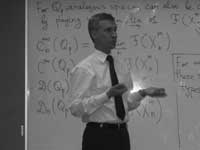The second one is the unpublished paper “Cohomology determinants and reciprocity laws : number field case” by Mikhail Kapranov and A. Smirnov.

This paper features in blog-posts at the Arcadian Functor, in John Baez’ Weekly Finds and in yesterday’s post at Noncommutative Geometry.

You can download every single page (of 15) as a separate file from here. But, in order to help spreading the Fun-gospel, I’ve made these scans into a single PDF-file which you can download as a 2.6 Mb PDF. In the introduction they say :

“First of all, it is an old idea to interpret combinatorics of finite sets as the $q \rightarrow 1$ limit of linear algebra over the finite field $\mathbb{F}_q$. This had lead to frequent consideration of the folklore object $\mathbb{F}_1$, the “field with one element”, whose vector spaces are just sets. One can postulate, of course, that $\mathbf{spec}(\mathbb{F}_1)$ is the absolute point, but the real problem is to develop non-trivial consequences of this point of view.”

They manage to deduce higher reciprocity laws in class field theory within the theory of $\mathbb{F}_1$ and its field extensions $\mathbb{F}_{1^n}$. But first, let us explain how they define linear algebra over these absolute fields.

Here is a first principle : in doing linear algebra over these fields, there is no additive structure but only scalar multiplication by field elements. So, what are vector spaces over the field with one element? Well, as scalar multiplication with 1 is just the identity map, we have that a vector space is just a set. Linear maps are just set-maps and in particular, a linear isomorphism of a vector space onto itself is a permutation of the set. That is, linear algebra over $\mathbb{F}_1$ is the same as combinatorics of (finite) sets.

A vector space over $\mathbb{F}_1$ is just a set; the dimension of such a vector space is the cardinality of the set. The general linear group $GL_n(\mathbb{F}_1)$ is the symmetric group $S_n$, the identification via permutation matrices (having exactly one 1 in every row and column)

Some people prefer to view an $\mathbb{F}_1$ vector space as a pointed set, the special element being the ‘origin’ $0$ but as $\mathbb{F}_1$ doesnt have a zero, there is also no zero-vector. Still, in later applications (such as defining exact sequences and quotient spaces) it is helpful to have an origin. So, let us denote for any set $S$ by $S^{\bullet} = S \cup { 0 }$. Clearly, linear maps between such ‘extended’ spaces must be maps of pointed sets, that is, sending $0 \rightarrow 0$.

The field with one element $\mathbb{F}_1$ has a field extension of degree n for any natural number n which we denote by $\mathbb{F}_{1^n}$ and using the above notation we will define this field as :

$\mathbb{F}_{1^n} = \mu_n^{\bullet}$ with $\mu_n$ the group of all n-th roots of unity. Note that if we choose a primitive n-th root $\epsilon_n$, then $\mu_n \simeq C_n$ is the cyclic group of order n.

Now what is a vector space over $\mathbb{F}_{1^n}$? Recall that we only demand units of the field to act by scalar multiplication, so each ‘vector’ $\vec{v}$ determines an n-set of linear dependent vectors $\epsilon_n^i \vec{v}$. In other words, any $\mathbb{F}_{1^n}$-vector space is of the form $V^{\bullet}$ with $V$ a set of which the group $\mu_n$ acts freely. Hence, $V$ has $N=d.n$ elements and there are exactly $d$ orbits for the action of $\mu_n$ by scalar multiplication. We call $d$ the dimension of the vectorspace and a basis consists in choosing one representant for every orbits. That is, $~B = { b_1,\ldots,b_d }$ is a basis if (and only if) $V = { \epsilon_n^j b_i~:~1 \leq i \leq d, 1 \leq j \leq n }$.

So, vectorspaces are free $\mu_n$-sets and hence linear maps $V^{\bullet} \rightarrow W^{\bullet}$ is a $\mu_n$-map $V \rightarrow W$. In particular, a linear isomorphism of $V$, that is an element of $GL_d(\mathbb{F}_{1^n})$ is a $\mu_n$ bijection sending any basis element $b_i \rightarrow \epsilon_n^{j(i)} b_{\sigma(i)}$ for a permutation $\sigma \in S_d$.

An $\mathbb{F}_{1^n}$-vectorspace $V^{\bullet}$ is a free $\mu_n$-set $V$ of $N=n.d$ elements. The dimension $dim_{\mathbb{F}_{1^n}}(V^{\bullet}) = d$ and the general linear group $GL_d(\mathbb{F}_{1^n})$ is the wreath product of $S_d$ with $\mu_n^{\times d}$, the identification as matrices with exactly one non-zero entry (being an n-th root of unity) in every row and every column.

This may appear as a rather sterile theory, so let us give an extremely important example, which will lead us to our second principle for developing absolute linear algebra.

Let $q=p^k$ be a prime power and let $\mathbb{F}_q$ be the finite field with $q$ elements. Assume that $q \cong 1~mod(n)$. It is well known that the group of units $\mathbb{F}_q^{\ast}$ is cyclic of order $q-1$ so by the assumption we can identify $\mu_n$ with a subgroup of $\mathbb{F}_q^{\ast}$.

Then, $\mathbb{F}_q = (\mathbb{F}_q^{\ast})^{\bullet}$ is an $\mathbb{F}_{1^n}$-vectorspace of dimension $d=\frac{q-1}{n}$. In other words, $\mathbb{F}_q$ is an $\mathbb{F}_{1^n}$-algebra. But then, any ordinary $\mathbb{F}_q$-vectorspace of dimension $e$ becomes (via restriction of scalars) an $\mathbb{F}_{1^n}$-vector space of dimension $\frac{e(q-1)}{n}$.

Next time we will introduce more linear algebra definitions (including determinants, exact sequences, direct sums and tensor products) in the realm the absolute fields $\mathbb{F}_{1^n}$ and remarkt that we have to alter the known definitions as we can only use the scalar-multiplication. To guide us, we have the second principle : all traditional results of linear algebra over $\mathbb{F}_q$ must be recovered from the new definitions under the vector-space identification $\mathbb{F}_q = (\mathbb{F}_q^{\ast})^{\bullet} = \mathbb{F}_{1^n}$ when $n=q-1$. (to be continued)

There are only a handful of human activities where one goes to extraordinary lengths to keep a dream alive, in spite of overwhelming evidence : religion, theoretical physics, supporting the Belgian football team and … mathematics.

In recent years several people spend a lot of energy looking for properties of an elusive object : the field with one element $\mathbb{F}_1$, or in French : “F-un”. The topic must have reached a level of maturity as there was a conference dedicated entirely to it : NONCOMMUTATIVE GEOMETRY AND GEOMETRY OVER THE FIELD WITH ONE ELEMENT.

In this series I’d like to find out what the fuss is all about, why people would like it to exist and what it has to do with noncommutative geometry. However, before we start two remarks :

The field $\mathbb{F}_1$ does not exist, so don’t try to make sense of sentences such as “The ‘field with one element’ is the free algebraic monad generated by one constant (p.26), or the universal generalized ring with zero (p.33)” in the wikipedia-entry. The simplest proof is that in any (unitary) ring we have $0 \not= 1$ so any ring must contain at least two elements. A more highbrow version : the ring of integers $\mathbb{Z}$ is the initial object in the category of unitary rings, so it cannot be an algebra over anything else.

The second remark is that several people have already written blog-posts about $\mathbb{F}_1$. Here are a few I know of : David Corfield at the n-category cafe and at his old blog, Noah Snyder at the secret blogging seminar, Kea at the Arcadian functor, AC and K. Consani at Noncommutative geometry and John Baez wrote about it in his weekly finds.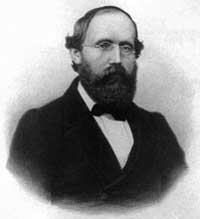The dream we like to keep alive is that we will prove the Riemann hypothesis one fine day by lifting Weil’s proof of it in the case of curves over finite fields to rings of integers.

Even if you don’t know a word about Weil’s method, if you think about it for a couple of minutes, there are two immediate formidable problems with this strategy.

For most people this would be evidence enough to discard the approach, but, we mathematicians have found extremely clever ways for going into denial.

The first problem is that if we want to think of $\mathbf{spec}(\mathbb{Z})$ (or rather its completion adding the infinite place) as a curve over some field, then $\mathbb{Z}$ must be an algebra over this field. However, no such field can exist…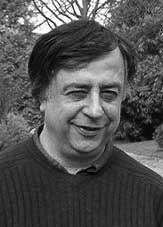No problem! If there is no such field, let us invent one, and call it $\mathbb{F}_1$. But, it is a bit hard to do geometry over an illusory field. Christophe Soule succeeded in defining varieties over $\mathbb{F}_1$ in a talk at the 1999 Arbeitstagung and in a more recent write-up of it : Les varietes sur le corps a un element.

We will come back to this in more detail later, but for now, here’s the main idea. Consider an existent field $k$ and an algebra $k \rightarrow R$ over it. Now study the properties of the functor (extension of scalars) from $k$-schemes to $R$-schemes. Even if there is no morphism $\mathbb{F}_1 \rightarrow \mathbb{Z}$, let us assume it exists and define $\mathbb{F}_1$-varieties by requiring that these guys should satisfy the properties found before for extension of scalars on schemes defined over a field by going to schemes over an algebra (in this case, $\mathbb{Z}$-schemes). Roughly speaking this defines $\mathbb{F}_1$-schemes as subsets of points of suitable $\mathbb{Z}$-schemes.

But, this is just one half of the story. He adds to such an $\mathbb{F}_1$-variety extra topological data ‘at infinity’, an idea he attributes to J.-B. Bost. This added feature is a $\mathbb{C}$-algebra $\mathcal{A}_X$, which does not necessarily have to be commutative. He only writes : “Par ignorance, nous resterons tres evasifs sur les proprietes requises sur cette $\mathbb{C}$-algebre.”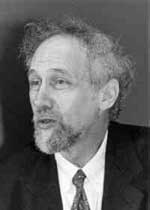The algebra $\mathcal{A}_X$ originates from trying to bypass the second major obstacle with the Weil-Riemann-strategy. On a smooth projective curve all points look similar as is clear for example by noting that the completions of all local rings are isomorphic to the formal power series $k[[x]]$ over the basefield, in particular there is no distinction between ‘finite’ points and those lying at ‘infinity’.

The completions of the local rings of points in $\mathbf{spec}(\mathbb{Z})$ on the other hand are completely different, for example, they have residue fields of different characteristics… Still, local class field theory asserts that their quotient fields have several common features. For example, their Brauer groups are all isomorphic to $\mathbb{Q}/\mathbb{Z}$. However, as $Br(\mathbb{R}) = \mathbb{Z}/2\mathbb{Z}$ and $Br(\mathbb{C}) = 0$, even then there would be a clear distinction between the finite primes and the place at infinity…

Alain Connes came up with an extremely elegant solution to bypass this problem in Noncommutative geometry and the Riemann zeta function. He proposes to replace finite dimensional central simple algebras in the definition of the Brauer group by AF (for Approximately Finite dimensional)-central simple algebras over $\mathbb{C}$. This is the origin and the importance of the Bost-Connes algebra.

We will come back to most of this in more detail later, but for the impatient, Connes has written a paper together with Caterina Consani and Matilde Marcolli Fun with $\mathbb{F}_1$ relating the Bost-Connes algebra to the field with one element.